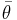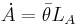# Difference between revisions of "Romer model"

The Romer model is a long-run economic growth model.

## Variables in the model

Name Variable Unit Set of possible values Rival input? Variable type Notes
Rate of discovery of new ideas$\bar\theta$
Technology A Endogenous
Number of people trying to discover new ideas$L_A$

## Mathematical formalism

In addition to the equations for the Solow–Swan model, there is an equation describing the discovery of new ideas (Jones & Vollrath, p100):$\dot A = \bar \theta L_A$